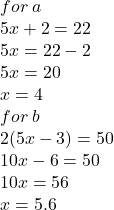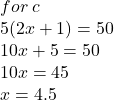## Figure out x for the following: a) 5x + 2 = 22 b) 2(5x – 3) = 50 c) 5(2x + 1) = 50

Question

Figure out x for the following:
a) 5x + 2 = 22
b) 2(5x – 3) = 50
c) 5(2x + 1) = 50

in progress 0
6 months 2021-08-04T10:51:16+00:00 2 Answers 6 views 0

4, 5.6, 4.5

Step-by-step explanation:

a:

5x + 2 = 22

5x = 20

x = 4

b:

2(5x-3) = 50

10x – 6 = 50

10x = 56

x = 5.6

c:

5(2x + 1) = 50

10x + 5 = 50

10x = 45

x = 4.5

2. Step-by-step explanation: